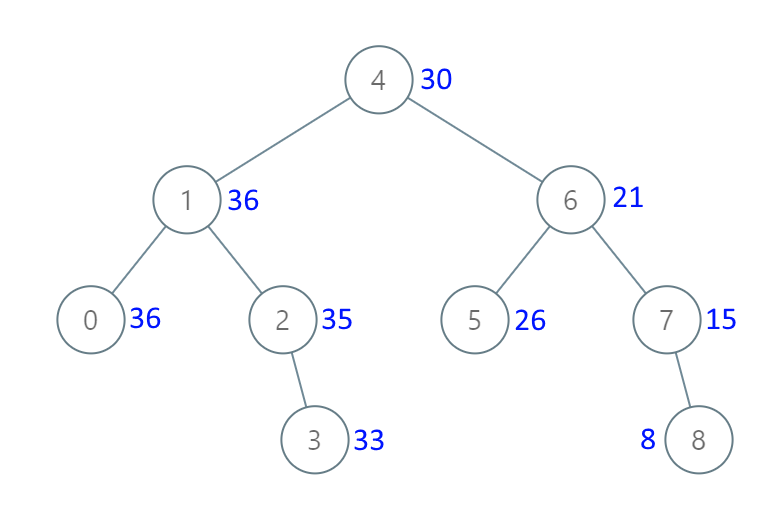# 1038. Binary Search Tree to Greater Sum Tree

Medium
Given the `root` of a Binary Search Tree (BST), convert it to a Greater Tree such that every key of the original BST is changed to the original key plus the sum of all keys greater than the original key in BST.
As a reminder, a binary search tree is a tree that satisfies these constraints:
• The left subtree of a node contains only nodes with keys less than the node's key.
• The right subtree of a node contains only nodes with keys greater than the node's key.
• Both the left and right subtrees must also be binary search trees.
Example 1:Input: root = [4,1,6,0,2,5,7,null,null,null,3,null,null,null,8]
Output:
[30,36,21,36,35,26,15,null,null,null,33,null,null,null,8]
Example 2:
Input: root = [0,null,1]
Output:
[1,null,1]
Constraints:
• The number of nodes in the tree is in the range `[1, 100]`.
• `0 <= Node.val <= 100`
• All the values in the tree are unique.
Note: This question is the same as 538: https://leetcode.com/problems/convert-bst-to-greater-tree/

### 解題

Runtime: 0 ms, faster than 100%
Memory Usage: 2.3 MB, less than 60.47%
/**
* Definition for a binary tree node.
* type TreeNode struct {
* Val int
* Left *TreeNode
* Right *TreeNode
* }
*/
func bstToGst(root *TreeNode) *TreeNode {
num := 0
var helper func(*TreeNode)
helper = func(root *TreeNode) {
if root == nil { return }
helper(root.Right)
num += root.Val
root.Val = num
helper(root.Left)
}
helper(root)
return root
}